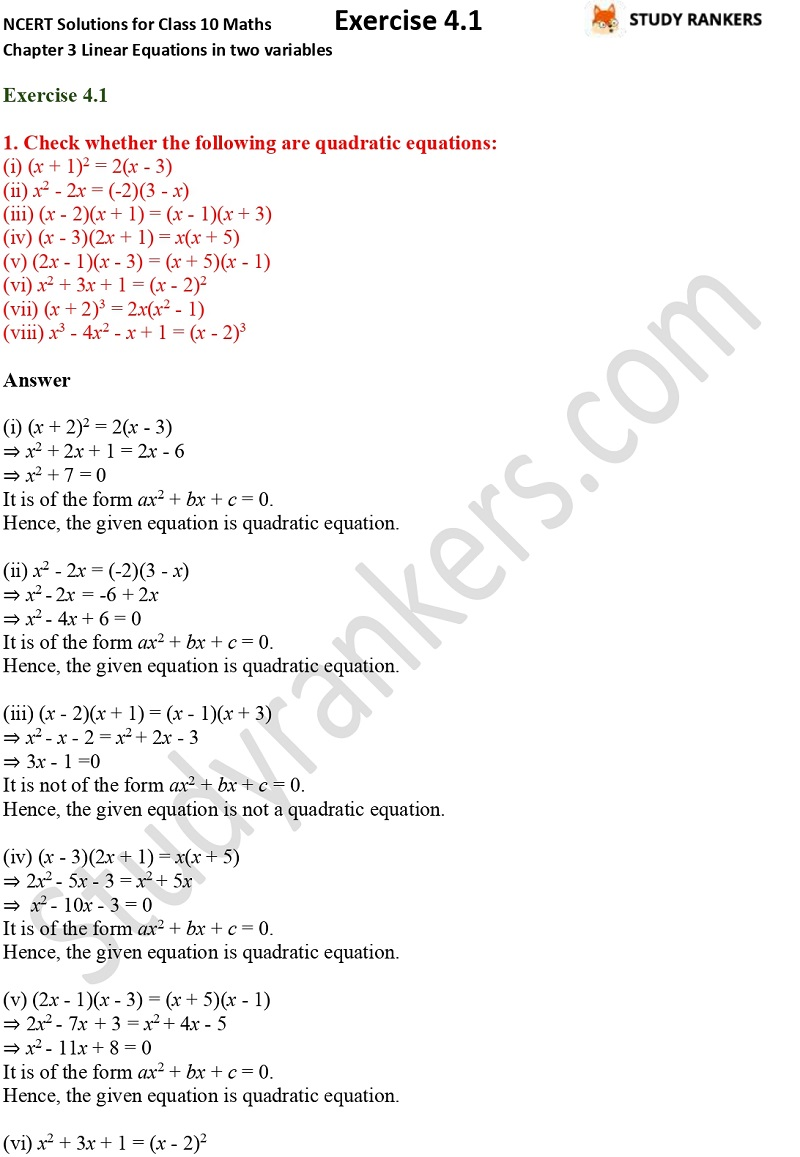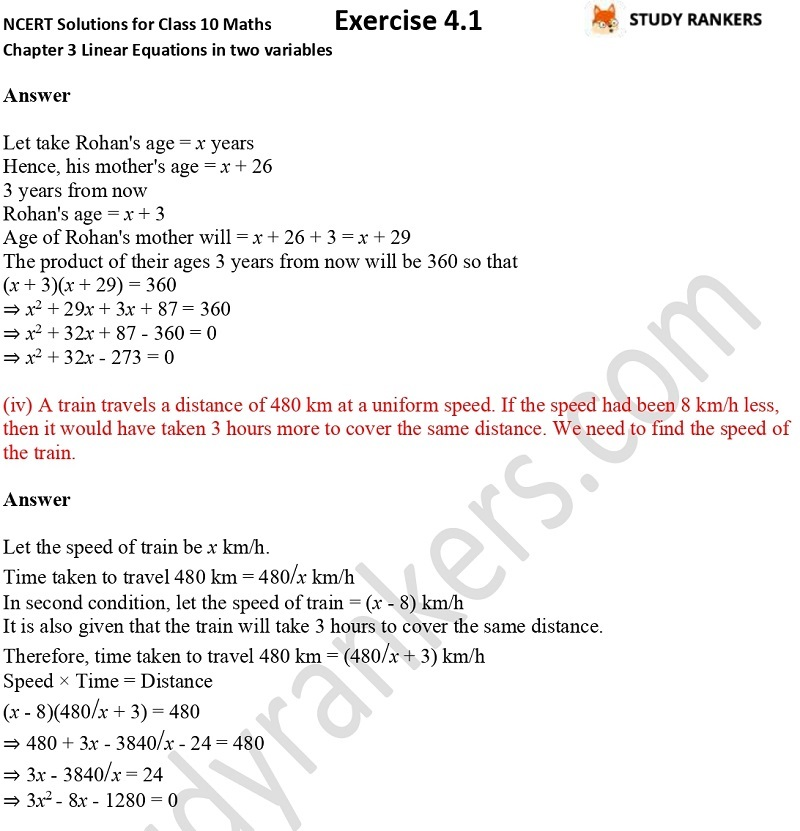>

## NCERT Solutions for Class 10 Maths Chapter 4 Quadratic Equations Exercise 4.1

In this page, you will find NCERT Solutions for Class 10 Maths Chapter 4 Quadratic Equations Exercise 4.1 which are updated as per the latest NCERT Books 2019-20. These will help you in knowing the concepts to solve questions. These CBSE NCERT solutions are helpful in completing your homework and improving your marks in the exams.

There are total main questions in this exercise 4.1. In the first you have to check given equations are quadratic equations or not and in the other you have represent the given situations in the form of quadratic equations.